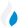•Trade Pi
•Trade
•Exchange
•Trade Pi
•Trade
•Exchange
\$1.11T
Total marketcap
\$58.72B
Total volume
40.14%
BTC dominance
•Trade Pi
•Trade
•Exchange

# DAI to VOLT Exchange Rate - 1 Dai in Asgardian Aereus

0
• BTC 0.000043
• ETH 0.0006
Vol [24h]
\$0

## dai to volt converter

Exchange Pair Price 24h volume

## DAI/VOLT Exchange Rate Overview

Name Ticker Price % 24h 24h high 24h low 24h volume
Dai dai \$1 0.1792% \$1 \$0.9982 \$128.36M
Asgardian Aereus volt \$2.3 -0.0673% \$0 \$0 \$20.04

Selling 1 DAI you get 0 Asgardian Aereus volt.

Dai Mar 13, 2020 had the highest price, at that time trading at its all-time high of \$1.22.

1059 days have passed since then, and now the price is 81.30% of the maximum.

Based on the table data, the DAI vs VOLT exchange volume is \$0.

Using the calculator/converter on this page, you can make the necessary calculations with a pair of Dai/Asgardian Aereus.

## Q&A

### What is the current DAI to VOLT exchange rate?

Right now, the DAI/VOLT exchange rate is 0.

### What has been the Dai to Asgardian Aereus trading volume in the last 24 hours?

Relying on the table data, the Dai to Asgardian Aereus exchange volume is \$0.

### How can I calculate the amount of VOLT? / How do I convert my Dai to Asgardian Aereus?

You can calculate/convert VOLT from Dai to Asgardian Aereus converter. Also, you can select other currencies from the drop-down list.

## DAI to VOLT Сonversion Table

DAI VOLT
0.5 DAI = 0 VOLT
1 DAI = 0 VOLT
2 DAI = 0 VOLT
3 DAI = 0 VOLT
5 DAI = 0 VOLT
10 DAI = 0 VOLT
20 DAI = 0 VOLT
50 DAI = 0 VOLT
200 DAI = 0 VOLT
2000 DAI = 0 VOLT
20000 DAI = 0 VOLT
200000 DAI = 0 VOLT
2000000 DAI = 0 VOLT